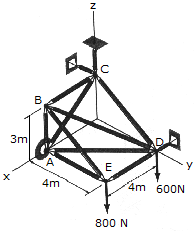# Engineering Mechanics - Equilibrium of a Rigid Body - Discussion

### Discussion :: Equilibrium of a Rigid Body - General Questions (Q.No.4)

4.The space truss is supported by a ball-and-socket joint at A and short links, two at C and one at D. Determine the x, y, z components of reaction at A and the force in each link.

 [A]. Ax = -1.050 kN, Ay = 1.050 kN, Az = 0.800 kN, Cy = -1.050 kN, Cz = 0.600 kN, Dx = 1.050 kN [B]. Ax = -1.400 kN, Ay = 1.400 kN, Az = 0.800 kN, Cy = -1.400 kN, Cz = 0.600 kN, Dx = 1.400 kN [C]. Ax = -2.49 kN, Ay = 1.867 kN, Az = 0.800 kN, Cy = -2.49 kN, Cz = 0.600 kN, Dx = 2.49 kN [D]. Ax = -1.867 kN, Ay = 1.867 kN, Az = 0.800 kN, Cy = -1.867 kN, Cz = 0.600 kN, Dx = 1.867 kN

Explanation:

No answer description available for this question.